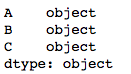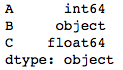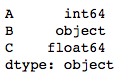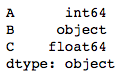# Change Data Type for one or more columns in Pandas Dataframe

Let’s see the different ways of changing Data Type for one or more columns in Pandas Dataframe.

Method #1: Using DataFrame.astype()

We can pass any Python, Numpy or Pandas datatype to change all columns of a dataframe to that type, or we can pass a dictionary having column names as keys and datatype as values to change type of selected columns.

 `# importing pandas as pd ` `import` `pandas as pd ` ` `  `# sample dataframe ` `df ``=` `pd.DataFrame({ ` `    ``'A'``: [``1``, ``2``, ``3``, ``4``, ``5``], ` `    ``'B'``: [``'a'``, ``'b'``, ``'c'``, ``'d'``, ``'e'``], ` `    ``'C'``: [``1.1``, ``'1.0'``, ``'1.3'``, ``2``, ``5``] }) ` ` `  `# converting all columns to string type ` `df ``=` `df.astype(``str``) ` `print``(df.dtypes) `

Output:`# importing pandas as pd ` `import` `pandas as pd ` ` `  `# sample dataframe ` `df ``=` `pd.DataFrame({ ` `    ``'A'``: [``1``, ``2``, ``3``, ``4``, ``5``], ` `    ``'B'``: [``'a'``, ``'b'``, ``'c'``, ``'d'``, ``'e'``], ` `    ``'C'``: [``1.1``, ``'1.0'``, ``'1.3'``, ``2``, ``5``] }) ` ` `  `# using dictionary to convert specific columns ` `convert_dict ``=` `{``'A'``: ``int``, ` `                ``'C'``: ``float` `               ``} ` ` `  `df ``=` `df.astype(convert_dict) ` `print``(df.dtypes) `

Output:Method #2: Using DataFrame.apply()

We can pass pandas.to_numeric, pandas.to_datetime and pandas.to_timedelta as argument to `apply()` function to change the datatype of one or more columns to numeric, datetime and timedelta respectively.

 `# importing pandas as pd ` `import` `pandas as pd ` ` `  `# sample dataframe ` `df ``=` `pd.DataFrame({ ` `    ``'A'``: [``1``, ``2``, ``3``, ``'4'``, ``'5'``], ` `    ``'B'``: [``'a'``, ``'b'``, ``'c'``, ``'d'``, ``'e'``], ` `    ``'C'``: [``1.1``, ``'2.1'``, ``3.0``, ``'4.1'``, ``'5.1'``] }) ` ` `  `# using apply method ` `df[[``'A'``, ``'C'``]] ``=` `df[[``'A'``, ``'C'``]].``apply``(pd.to_numeric) ` `print``(df.dtypes) `

Output:Method #3: Using DataFrame.infer_objects()
This method attempts soft-conversion by inferring data type of ‘object’-type columns. Non-object and unconvertible columns are left unchanged.

 `# importing pandas as pd ` `import` `pandas as pd ` ` `  `# sample dataframe ` `df ``=` `pd.DataFrame({ ` `    ``'A'``: [``1``, ``2``, ``3``, ``4``, ``5``], ` `    ``'B'``: [``'a'``, ``'b'``, ``'c'``, ``'d'``, ``'e'``], ` `    ``'C'``: [``1.1``, ``2.1``, ``3.0``, ``4.1``, ``5.1``] ` `     ``}, dtype ``=``'object'``) ` ` `  `# converting datatypes ` `df ``=` `df.infer_objects() ` `print``(df.dtypes) `

Output:Attention geek! Strengthen your foundations with the Python Programming Foundation Course and learn the basics.

To begin with, your interview preparations Enhance your Data Structures concepts with the Python DS Course.

My Personal Notes arrow_drop_upCheck out this Author's contributed articles.

If you like GeeksforGeeks and would like to contribute, you can also write an article using contribute.geeksforgeeks.org or mail your article to contribute@geeksforgeeks.org. See your article appearing on the GeeksforGeeks main page and help other Geeks.

Please Improve this article if you find anything incorrect by clicking on the "Improve Article" button below.

Article Tags :

2

Please write to us at contribute@geeksforgeeks.org to report any issue with the above content.